# Python | Monitor hard-disk health using smartmontools

• Last Updated : 25 Jun, 2019

Smartmontools, an acronym for ‘S.M.A.R.T monitoring’ tools is a package that is used to control and monitor computer storage systems using S.M.A.R.T. (Self-Monitoring, Analysis and Reporting Technology) system built into most modern (P)ATA, Serial ATA, SCSI/SAS devices.
It contains 2 utility programs: smartctl and smartd. These utilities give warnings or alerts of disk degradation and failure.
`smartmontools `can be used in any Unix/Linux based operating systems. It allows us to run various tests to check the health of the HDD or SSD in your system.

## S.M.A.R.T

Most modern hard drives use S.M.A.R.T. (Self-Monitoring, Analysis, and Reporting Technology) to assess their condition to determine if something is wrong with the device. This allows the user to view the hard drive’s SMART data and take necessary actions to repair or replace the device.

In this article, we will explore smartmontools and retrieve information on the HDDs and SSD in the system. We will also write a python script to parse the output of smartmontools and store the outputs in an excel sheet.

## Installations –

```sudo pip3 install pandas
sudo apt-get install smartmontools ```

Once smartmontools has been installed, we can use the terminal or command line to obtain details of the hard drives.

## Device information –

To check whether your device supports SMART monitoring and to obtain other information such as device model, capacity, serial number, etc, we use the following command:

`sudo smartctl -i /dev/sda`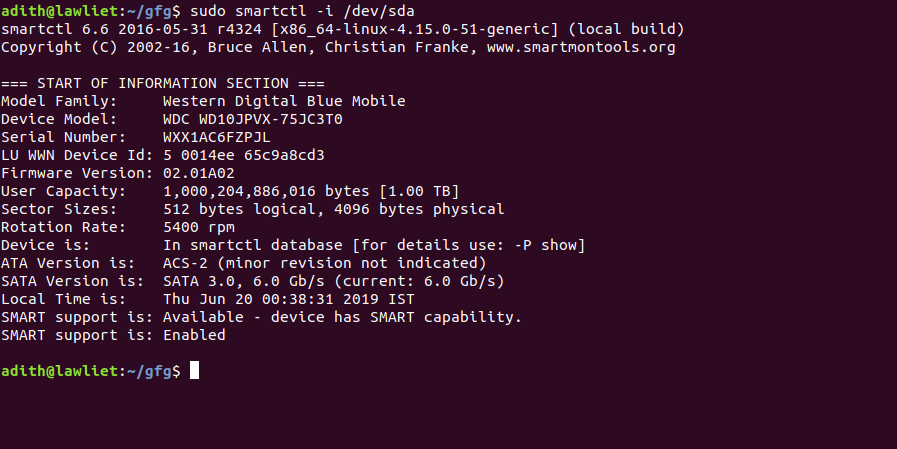If it is not enabled, the following command enables SMART monitoring:

`sudo smartctl -s on /dev/sda`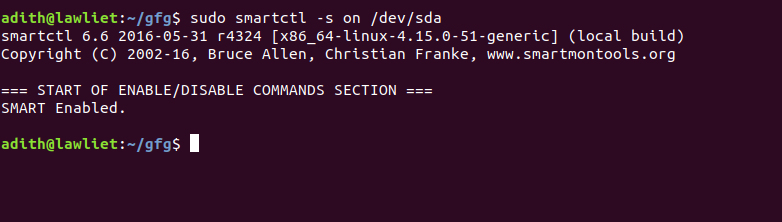## Checking the health of the device

To display the overall health of the disk, we use the following command:

`sudo smartctl -H /dev/sda`

This displays the status of your hard drive. If it displays any errors, then your hard drive might be experiencing some problems and you should consider backing up your data.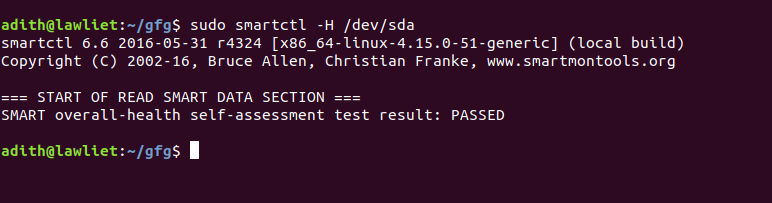## Tests –

To run a short test :

`sudo smartctl --test=short /dev/sda`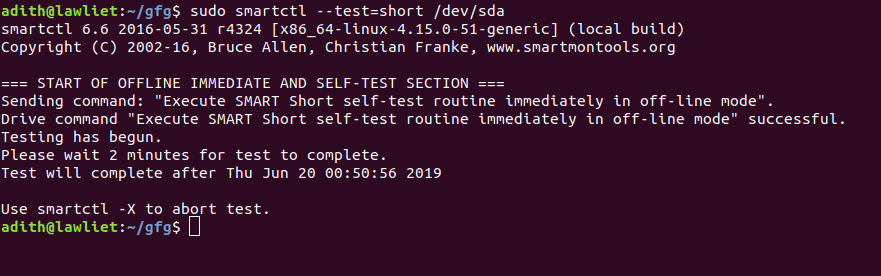The goal of the short test is the rapid identification of a defective hard drive. Therefore, the maximum run time for the short test is 2 min.

To run a long test:

`sudo smartctl --test=long /dev/sda`

Long tests also identify defects but here, there is no time restriction. The test is more thorough.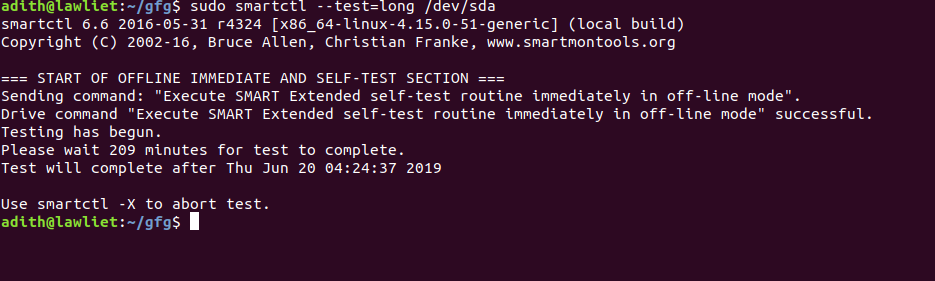To check the results of the test:

`sudo smartctl -l selftest /dev/sda`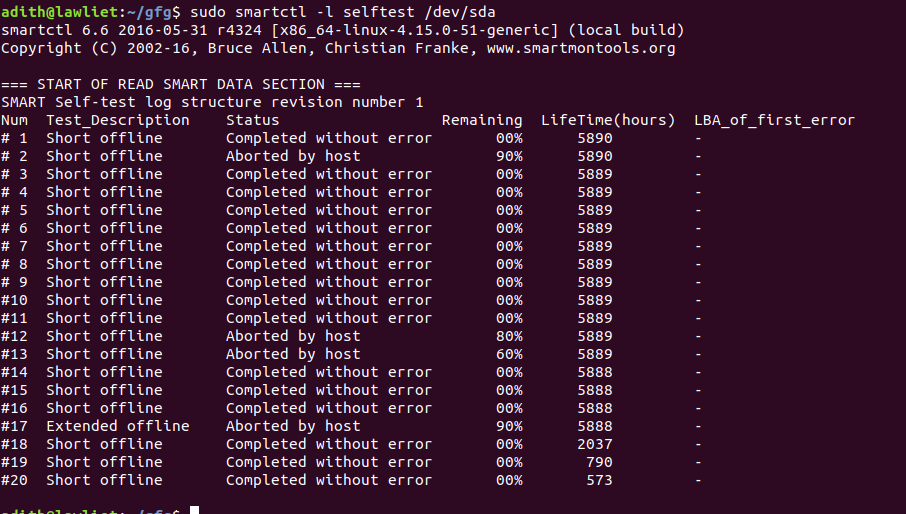## An example –

We can use Python to automate this process and generate a report. For this, we shall use Pandas to store the result in excel sheets and the os module to run the commands.

 `# importing libraries``import` `os``import` `pandas as pd``from` `pandas ``import` `ExcelWriter`` ` `# class to hold all the``# details about the device``class` `Device():`` ` `    ``def` `__init__(``self``):`` ` `        ``self``.device_name ``=` `None``        ``self``.info ``=` `{}``        ``self``.results ``=` `[]`` ` `    ``# get the details of the device``    ``def` `get_device_name(``self``):`` ` `        ``cmd ``=` `'smartctl --scan'`` ` `        ``data ``=` `os.popen(cmd)``        ``res ``=` `data.read()``        ``temp ``=` `res.split(``' '``)`` ` `        ``temp ``=` `temp[``0``].split(``'/'``)``        ``name ``=` `temp[``2``]``        ``self``.device_name ``=` `name`` ` ` ` `    ``# get the device info (sda or sdb)``    ``def` `get_device_info(``self``):`` ` `        ``cmd ``=` `'smartctl -i /dev/'` `+` `self``.device_name`` ` `        ``data ``=` `os.popen(cmd)`` ` `        ``res ``=` `data.read().splitlines()`` ` `        ``device_info ``=` `{}`` ` `        ``for` `i ``in` `range``(``4``, ``len``(res) ``-` `1``):``            ``line ``=` `res[i]``            ``temp ``=` `line.split(``':'``)``            ``device_info[temp[``0``]] ``=` `temp[``1``]`` ` `        ``self``.info ``=` `device_info`` ` `    ``# save the results as an excel file``    ``def` `save_to_excel(``self``):`` ` `        ``try``:``            ``os.mkdir(``'outputs'``)``        ``except``(Exception):``            ``pass`` ` `        ``os.chdir(``'outputs'``)`` ` `        ``col1 ``=` `list``(``self``.info.keys())``        ``col2 ``=` `list``(``self``.info.values())`` ` `        ``output ``=` `pd.DataFrame()``        ``output[``'Name'``] ``=` `col1``        ``output[``'Info'``] ``=` `col2`` ` `        ``writer ``=` `ExcelWriter(``'Device_info.xlsx'``)``        ``output.to_excel(writer, ``'Info_report'``, index ``=` `False``)`` ` `        ``workbook ``=` `writer.book``        ``worksheet ``=` `writer.sheets[``'Info_report'``]`` ` `        ``# Account info columns``        ``worksheet.set_column(``'A:A'``, ``35``)``        ``# State column``        ``worksheet.set_column(``'B:B'``, ``55``)``        ``# Post code``        ``# worksheet.set_column('F:F', 10)``        ``writer.save()``        ``os.chdir(``'..'``)`` ` `    ``# function to check the health``    ``# of the device``    ``def` `check_device_health(``self``):`` ` `        ``cmd ``=` `'smartctl -H /dev/'` `+` `self``.device_name`` ` `        ``data ``=` `os.popen(cmd).read()``        ``res ``=` `data.splitlines()``        ``health ``=` `res[``4``].split(``':'``)``        ``print``(health[``0``] ``+` `':'` `+` `health[``1``])`` ` ` ` `    ``# function to run the short test``    ``def` `run_short_test(``self``):`` ` `        ``cmd ``=` `'smartctl --test = short /dev/'` `+` `self``.device_name``        ``data ``=` `os.popen(cmd).read().splitlines()`` ` ` ` `    ``# function to get the results``    ``# of the test.``    ``def` `get_results(``self``):`` ` `        ``cmd ``=` `'smartctl -l selftest /dev/'` `+` `self``.device_name``        ``data ``=` `os.popen(cmd).read()``        ``res ``=` `data.splitlines()`` ` `        ``# stores the names of columns``        ``columns ``=` `res[``5``].split(``' '``)``        ``columns ``=` `' '``.join(columns)``        ``columns ``=` `columns.split()`` ` `        ``info ``=` `[columns]`` ` `        ``# iterate through the important``        ``# rows since 0-5 is not required``        ``for` `i ``in` `range``(``6``, ``len``(res)):``                 ` `            ``line ``=` `res[i]`` ` `            ``line ``=` `' '``.join(line.split())``            ``row ``=` `line.split(``' '``)``            ``info.append(row)`` ` `        ``# save the results``        ``self``.results ``=` `info`` ` ` ` `    ``# function to convert the ``    ``# results of the test to an``    ``# excel file and save it``    ``def` `save_results_to_excel(``self``):`` ` `        ``# create a folder to store outputs``        ``try``:``            ``os.mkdir(``'outputs'``)``        ``except``(Exception):``            ``pass`` ` `        ``os.chdir(``'outputs'``)`` ` `        ``# get the columns``        ``columns ``=` `self``.results[``0``]`` ` `        ``# create a dataframe to store``        ``# the result in excel``        ``outputs ``=` `pd.DataFrame()`` ` `        ``col1, col2, col3, col4 ``=` `[], [], [], []``         ` `        ``l ``=` `len``(``self``.results[``1``])`` ` `        ``# iterate through all the rows and store``        ``# it in the data frame``        ``for` `i ``in` `range``(``1``, ``len``(``self``.results) ``-` `1``):`` ` `            ``if``(``len``(``self``.results[i]) ``=``=` `l):``                ``col1.append(``' '``.join(``self``.results[i][``2``:``4``]))``                ``col2.append(``' '``.join(``self``.results[i][``4``:``7``]))``                ``col3.append(``self``.results[i][``7``])``                ``col4.append(``self``.results[i][``8``])            ``            ``else``:`` ` `                ``col1.append(``' '``.join(``self``.results[i][``1``:``3``]))``                ``col2.append(``' '``.join(``self``.results[i][``3``:``6``]))``                ``col3.append(``self``.results[i][``6``])``                ``col4.append(``self``.results[i][``7``])    `` ` `        ``# store the columns that we ``        ``# require in the data frame``        ``outputs[columns[``1``]] ``=` `col1``        ``outputs[columns[``2``]] ``=` `col2``        ``outputs[columns[``3``]] ``=` `col3``        ``outputs[columns[``4``]] ``=` `col4``         ` `        ``# an excel writer object to save as excel.``        ``writer ``=` `ExcelWriter(``'Test_results.xlsx'``)``     ` `        ``outputs.to_excel(writer, ``'Test_report'``, index ``=` `False``)`` ` `        ``# manipulating the dimensions of the columns``        ``# to make it more presentable.``        ``workbook ``=` `writer.book``        ``worksheet ``=` `writer.sheets[``'Test_report'``]`` ` `        ``worksheet.set_column(``'A:A'``, ``25``)``        ``worksheet.set_column(``'B:B'``, ``25``)``        ``worksheet.set_column(``'C:C'``, ``25``)``        ``worksheet.set_column(``'D:D'``, ``25``)`` ` `        ``# saving the file``        ``writer.save()`` ` `        ``os.chdir(``'..'``)`` ` ` ` `# driver function``if` `__name__ ``=``=` `'__main__'``:`` ` `    ``device ``=` `Device()``    ``device.get_device_name() ``    ``device.get_device_info()  ``    ``device.save_to_excel() ``    ``device.check_device_health()``    ``device.run_short_test()``    ``device.get_results()``    ``device.save_results_to_excel()`

Output :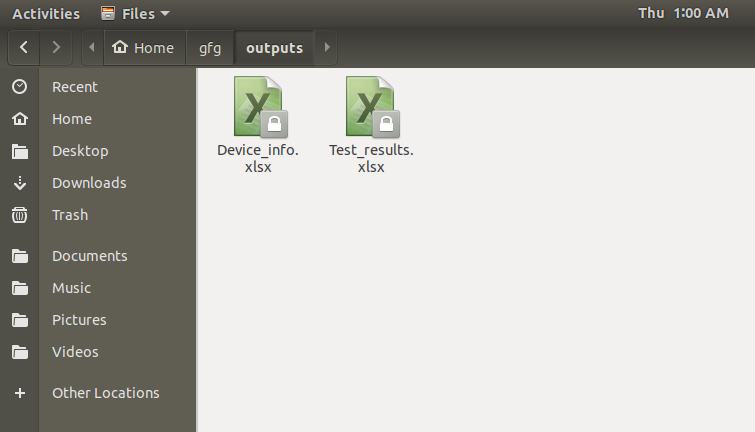Device Info: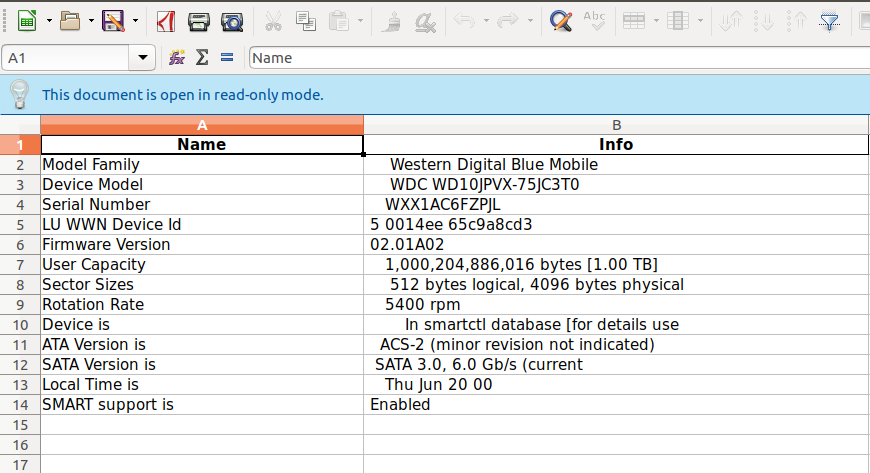Test results: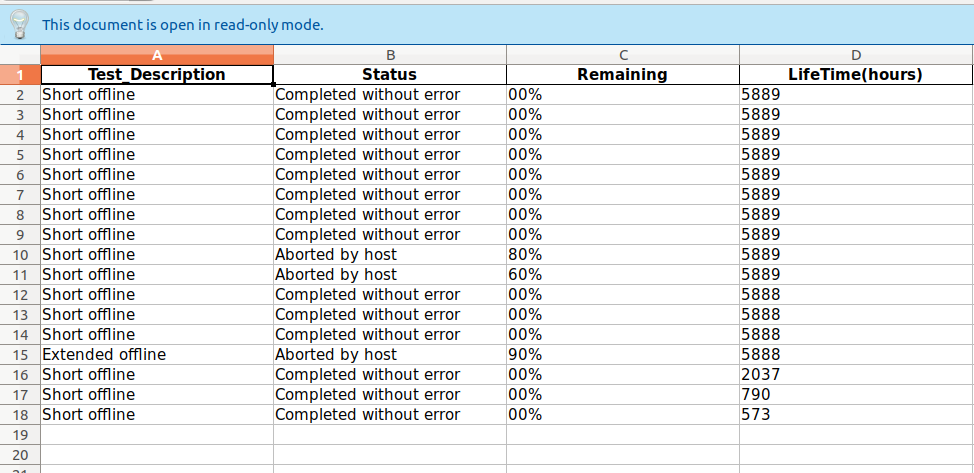My Personal Notes arrow_drop_up